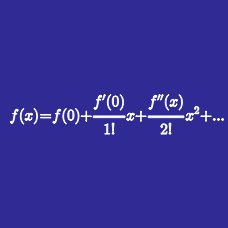Calculus

# Taylor Series - Error Bounds

Find the error bound for the $4^\text{th}$ degree polynomial of

$f(x)=\sin x ~\text{ centered at }~ x=0 ~\text{ on the interval }~ [0,2\pi].$

Find the error bound for the $4^\text{th}$ degree polynomial of

$f(x)=\cos x ~\text{ centered at }~ x=0 ~\text{ on the interval }~ [0,2\pi].$

What is the maximum possible error of the $10^\text{th}$ degree polynomial of

$f(x)=e^{x} ~\text{ centered at }~ x=0 ~\text{ on the interval }~ [-2,2]?$

What is the maximum possible error of the $1^\text{st}$ degree polynomial of

$f(x)=\sqrt{1+x} ~\text{ centered at }~ x=0 ~\text{ on the interval }~ [-0.01,0.01]?$

What is the maximum possible error of the $2^\text{nd}$ degree polynomial of

$f(x)=\ln(1+x) ~\text{ centered at }~ x=0 ~\text{ on the interval }~ [-0.1,0.1]?$

×